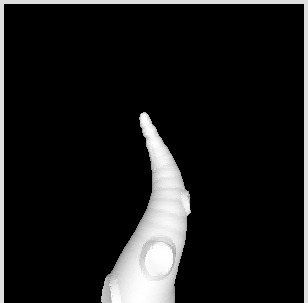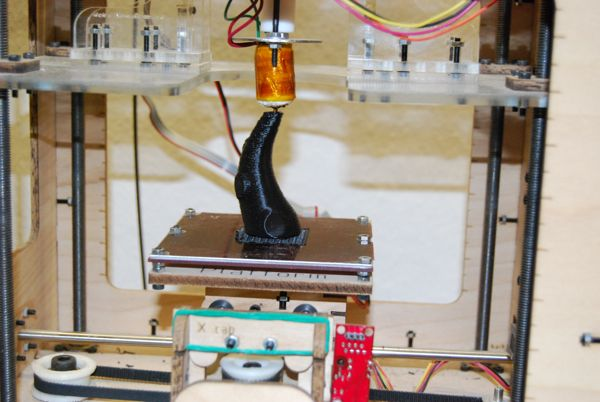## how to make a tentacle using processing and toxiclibs

the Toxiclibs are a very interesting collection of processing libraries. One of the libraries is called volumeutils and allows to use a volumetric space to model a 3D object.

I used it to generate a tentacle and export it as a stl file to make it printable on my 3D-printer.In the setup method i define a volumetric space containing 100x100x100 voxel, a iso surface and a Triangle mesh (which is needed to display the object and export it later)

``````gfx = new ToxiclibsSupport(this);
VolumetricSpace volume = new VolumetricSpaceArray( new Vec3D(100,200,100), 100, 100, 100 );
IsoSurface surface = new ArrayIsoSurface( volume );
mesh = new TriangleMesh();
``````

Then i define a volumentric brush - in this case a sphere and use it to draw a spiral while increasing the raduis of the brush-sphere.

``````VolumetricBrush brush = new RoundBrush( volume, 10 );
for ( int i=0; i < 20; i ++) {
brush.setSize( i*1.2 + 6 );
float x = cos( i * TWO_PI / 20 ) * 10;
float y = sin( i * TWO_PI / 20 ) * 10;
brush.drawAtAbsolutePos( new Vec3D(x,-25+i * 7,y), 1 );
}
``````

Then i use the brush to draw the suckers. First I draw a sphere a little bit outside the tentacle and then I draw a second sphere with a smaller raduis and a negative density to remove a section of a sphere and leave a dent.

``````for ( int i=4; i < 20; i+=4) {
brush.setSize( i/1.5+4 );

float x = cos( i * TWO_PI / 20 ) * (i*1.2+16);
float y = sin( i * TWO_PI / 20 ) * (i*1.2+16);

brush.drawAtAbsolutePos( new Vec3D(x,-25+i * 7,y), 1 );

brush.setSize( i/2+2 );
float x2 = cos( i * TWO_PI / 20  ) * (i*1.2+18);
float y2 = sin( i * TWO_PI / 20  ) * (i*1.2+18);
brush.drawAtAbsolutePos( new Vec3D(x2,-25+i * 7,y2), -1.4 );
}
``````

finally the mesh gets generated from the volumetric object.

``````volume.closeSides();
surface.reset();
surface.computeSurfaceMesh( mesh, .5 );
``````

to display the mesh i use the ToxiclibsSupport to generate the vertices in the draw method

``````beginShape(TRIANGLES);
gfx.mesh( mesh );
endShape();
``````

exporting the mesh to stl is simply a matter of calling

``````mesh.saveAsSTL( sketchPath( "tentacle.stl" ));
``````

Leave a response

1. Cool Stuff! I need to learn some processing. Need to do this stuff as well :)

2. hey! Your 3d printer... is it from the reprap project or somewhere else? cheers

3. Hi-

I'm playing with your example (thanks!) and it looks like the handedness between the Processing displayed images and the exported STL files is reversed.

I think the Z axis is flipped, as the exported STL appears upside-down from what is displayed in Processing. Did you resolve this?

-Rob A>

4. Answered my own question... Y axis, not Z (I keep forgetting up is Y in Processing).

I changed the save code to: void keyPressed() { if (key=='s') { mesh.flipYAxis(); mesh.saveAsSTL( sketchPath( "tentacle.stl" )); mesh.flipYAxis(); } }

and this now exports an STL file that looks exactly like what was displayed in Processing.

-Rob A>

5. hi ! was very helpful to me in order to keep going understanding how tx.libs works ! thanks ! dx